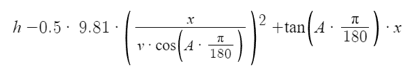# Projectile Intercept Math & Trigonometry

• VexCarido
VexCarido
Looking for some guidance on how to set up the equations for a projectile intercept given that you have perfect information about the target velocity, size and weather conditions in a 3D scenario, it's for an amateur videogame that I'm developing in my spare time

For simplicity sake let's assume there's no wind force and that target object is launched from coordinate (5,100,0) at a 55 degree angle along the x-axis, with initial velocity of 40 m/s - we have to launch to intercept the projectile with a delay of 2 second after the initial object is launched. Time of Flight for the object is 6.68 seconds. and we're launching from the origin coordinate (0,0,0)

Our initial projectile launch velocity range is 60 to 120 m/s and with a solution we should end up with a list of possible combinations of angles, and projectile speeds that would successfully intercept the target.

I know how to calculate the trajectory, as well as the horizontal/vertical range & time of flight for both objects I don't think this should be more difficult than rearranging a few components of the equations of motion, but I am at a loss as to how I should go about it exactly. Is there a swift and easy & efficient way to do this?

Homework Helper
Gold Member
Is there a swift and easy & efficient way to do this?
I don't think there is. You are not answering a homework problem with specific input parameters. You need a general solution given the ever-changing parameters that depend on the user is doing. You are probably looking for expressions for the projection angles (##\theta,~\phi##) of the interceptor given that its speed is fixed at ##v_0.## So you need to write some equations for the position of the enemy projectile and the interceptor as functions of time, take the difference (note that the acceleration term drops out), and see what must be true for that difference to be zero.

VexCarido
I don't think there is. You are not answering a homework problem with specific input parameters. You need a general solution given the ever-changing parameters that depend on the user is doing. You are probably looking for expressions for the projection angles (##\theta,~\phi##) of the interceptor given that its speed is fixed at ##v_0.## So you need to write some equations for the position of the enemy projectile and the interceptor as functions of time, take the difference (note that the acceleration term drops out), and see what must be true for that difference to be zero.
Figuring out the position of the target as a function of time is not the hard part, this can be done very simply by rearranging the 2D equation for the trajectory of a projectile to account for the added z axis.Or by implementing the Euler method to approximate the trajectory instead.

It's what I do after this that I need help with. To simplify the problem even further let's fix our own initial launch velocity to 80 m/s.

Shreya
Summary:: Need help to understand the math of projectile intercept for videogame

more difficult than rearranging a few components of the equations of motion, but I am at a loss as to how I should go about it exactly. Is there a swift and easy & efficient way to do this?
I think you will need to equate the coordinates of both the projectiles.
Equate the x(t) of 1st and second particle. And do the same with y(t). Put in the value of t.
You will get the angle of projection this way, using that you will get your other parameters.
A computer program would able to do this easily.
Sorry if I am wrong